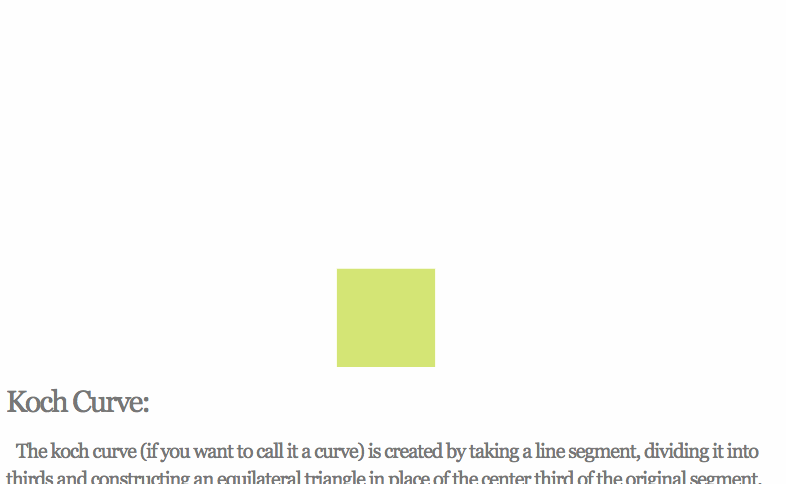swizec.com

#### Senior Mindset Book

Get promoted, earn a bigger salary, work for top companies

# Fractals in React

So… this started as an article about why recursion doesn't work in React. It looks like it works, then you `npm run build`, and it stops working.

Curious, right? Worth looking into, eh?

That's not the article you're getting. It started as that article, then I spent 3 hours building a Pythagoras tree fractal. It's 2:30am, and is my life even real?

Who the hell accidentally spends all night building fractals? Me… I guess.Pretty, innit? Built with React, and it's going to stop working when I `npm run build`. Still don't know why. I'll figure that out next week.

Here's how the Pythagoras tree works:

The construction of the Pythagoras tree begins with a square. Upon this square are constructed two squares, each scaled down by a linear factor of ½√2, such that the corners of the squares coincide pairwise. The same procedure is then applied recursively to the two smaller squares, ad infinitum.

?

That becomes four bullet points:

• 1 component called `<Pythagoras >`
• draws rectangle
• calculates props for next 2 rectangles
• `<Pythagoras><Pythagoras>`

Which turns into some 30 lines of code:

```import React from 'react';import { interpolateViridis } from 'd3-scale';.css-13aqjzy{display:inline-block;}
const Factor = .5*Math.sqrt(2);
const Pythagoras = ({ maxlvl, w, x, y, lvl, left, right }) => {    if (lvl > maxlvl || w < 1) {        return null;    }
const nextLeft = Factor*w,          nextRight = Factor*w,          d = nextLeft + nextRight + w,          A = 45,          B = 45;
let rotate = '';
if (left) {        rotate = `rotate(\${-A} 0 \${w})`;    }else if (right) {        rotate = `rotate(\${B} \${w} \${w})`;    }
return (        <g transform={`translate(\${x} \${y})="" \${rotate}`}="">            <rect width={w} height={w} x={0} y={0} style="{{fill:" interpolateviridis(lvl="" maxlvl)}}="">
<pythagoras w={nextLeft} x={w-nextLeft} y={-nextLeft} lvl={lvl+1} maxlvl={maxlvl} right="">
<pythagoras w={nextRight} x={0} y={-nextRight} lvl={lvl+1} maxlvl={maxlvl} left="">        </pythagoras></pythagoras></rect></g>    );};

export default Pythagoras;```

Beautiful. Let me explain.

`interpolateViridis` is a d3-scale that gives beautiful colors. Call it with an argument in `[0, 1]` and it returns a color.

`Factor` is the constant linear factor. We use it to calculate the sides of future rectangles.

`d` is the diameter of the triangle formed by the current square and two future squares. More on that later.

`A` and `B` are angles for each future rectangle. Set to 45 degrees statically.

## Then we start drawing.

If we're in a `left` rectangle, we set up a left rotation; if `right` then a right rotation. `rotate()` is an SVG transformation that rotates the current coordinate system.

To draw the rectangle, we:

• `translate` to `(x, y`), that means "move there"
• now our coordinate system is moved and rotate
• draw a rectangle at `(0, 0)`
• add two `<Pythagoras>` with new parameters

And that's how you build a fractal in React. It won't work in production, but it sure looks pretty on your localhost.The animation is done in App.js with a timer that updates the `maxlvl` prop every 500ms. Calling the root node of `Pythagoras` looks like this:

`<pythagoras  w={100}  x={320 - 50}  y={480 - 100}  lvl={0}  maxlvl="{this.state.currentMax}/"></pythagoras>`

Start `lvl` at `0` and set the `maxlvl`. Those are important. At `maxlvl` past 12 or 13, it stops working. It takes too much CPU power to ever render.

Yes, I tried. The naive algorithm isn't good enough. You could optimize by taking calculations out of recursion and preparing them in advance.

## The part I can't figure out

Look at Andrew Hoyer's Pythagoras tree. That thing is beautiful and flexible and dances like tree-shaped worm.?

I can't figure out how to calculate those angles and rectangle sizes. I know that using `.5` in the `Factor` is for `45` degree angles.

You can change the ratio by using a `.3` and `.7` factor for each side. Then it stops working with `45` degree angles yeah.Ok, that was expected. Since you know all the sides, you should be able to apply the Law of Sines to calculate the angle.

`const nextLeft = 0.3 * Factor * w,  nextRight = 0.7 * Factor * w,  d = nextLeft + nextRight + w,  A = Math.degrees(Math.asin(nextRight / d)),  B = Math.degrees(Math.asin(nextLeft / d));`I can't figure it out. I'm pretty sure I'm applying the Law of Sines correctly, but the numbers it throws out are wrong.

Halp ?

PS: Here's a paper that describes using Pythagoras trees as data structures. Sort of.

Created by Swizec with ❤️